Refer to our Texas Go Math Grade 2 Answer Key Pdf to score good marks in the exams. Test yourself by practicing the problems from Texas Go Math Grade 2 Lesson 7.5 Answer Key Find Sums for 4 Addends.

Explore

Show how you solved each problem.

FOR THE TEACHER • Read this problem and have children choose a way to solve it. Shelly counts 16 ants in her ant farm. Pedro counts 22 ants in his farm. Tara counts 14 ants in her farm. How many ants do the 3 children count? Repeat for another problem.
Shelly counts 16 ants in her ant farm.
Pedro counts 22 ants in his farm.
Tara counts 14 ants in her farm.
16 + 22 + 14 = 52
52 ants that the 3 children count

Math Talk
Mathematical Processes

Describe how you found the answer to the first problem.
16 + 22 + 14 = 52
Explanation:
The sum of 3 two digit numbers are added

Model and Draw

You can add digits within a column in any order. Add the ones first. Then add the tens.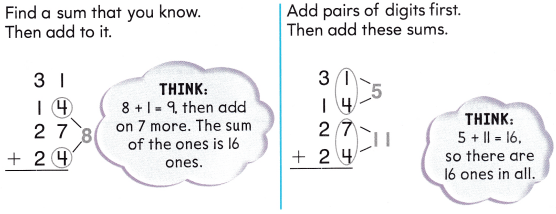Share and Show

Question 1.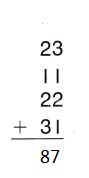Explanation:
The sum of 4 two digit numbers are added
23 + 11 + 22 + 31 = 87

Question 2.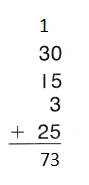Explanation:
The sum of 4 two digit numbers are added
30 + 15 + 3 + 25 = 73

Question 3.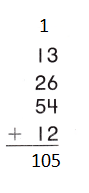Explanation:
The sum of 4 two digit numbers are added
13 + 26 + 54 + 12 = 105

Question 4.Explanation:
The sum of 4 two digit numbers are added
27 + 2 + 23 + 13 = 65

Question 5.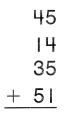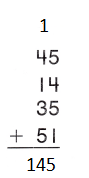45 + 14 + 35 + 51 = 145
Explanation:
The sum of 4 two digit numbers are added

Question 6.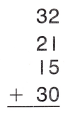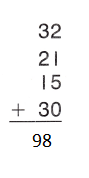Explanation:
The sum of 4 two digit numbers are added
32 + 21 + 15 + 30 = 98

Problem Solving

Question 7.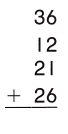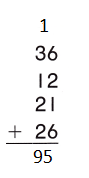Explanation:
The sum of 4 two digit numbers are added
36 + 12 + 21 + 26 = 95

Question 8.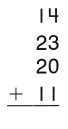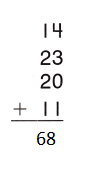Explanation:
The sum of 4 two digit numbers are added
14 + 23 + 20 + 11 = 68

Question 9.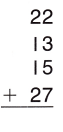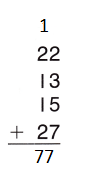Explanation:
The sum of 4 two digit numbers are added
22 + 13 + 15 + 27 = 77

Question 10.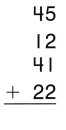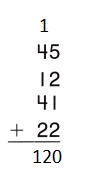Explanation:
The sum of 4 two digit numbers are added
45 + 12 + 41 + 22 = 120

Question 11.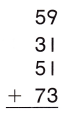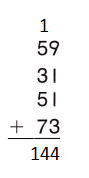Explanation:
The sum of 4 two digit numbers are added
59 + 31 + 51 + 3 = 144

Question 12.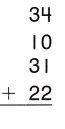Explanation:
The sum of 4 two digit numbers are added
34 + 10 + 31 + 22 = 97

Solve. Write or draw to explain.

Question 13.
H.O.T. Multi-Step Laney added four numbers which have a total of 128. She spilled some juice over one number. What is that number?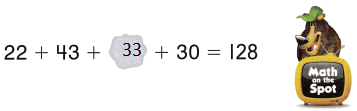Explanation:
He spilled the juice on number 33

Question 14.
H.O.T. Multi-Step Some friends need 100 shells for a project. Kate brings 21 shells, Paul brings 44 shells, and Noah brings 27 shells. How many more shells do they need?__________ more shells
8 more shells
Explanation:
Some friends need 100 shells for a project.
Kate brings 21 shells,
Paul brings 44 shells,
and Noah brings 27 shells.
21 + 44 + 27 = 92
100 – 92 = 8
8  more shells that they need

Question 15.
Multi-Step John sees a group of 47 butterflies, a group of 30 butterflies, a group of 55 butterflies, and a group of 26 butterflies. How many butterflies does he see?
(A) 168
(B) 146
(C) 158
Explanation:
John sees a group of 47 butterflies,
a group of 30 butterflies,
a group of 55 butterflies,
and a group of 26 butterflies.
47 + 30 + 55 + 26 = 158
158 butterflies that he see

Question 16.
Connect At the Kite Festival there are 19 blue kites, 35 green kites, 20 yellow kites, and 23 orange kites. How many kites are there?
(A) 94
(B) 97
(C) 87
Explanation:
At the Kite Festival there are 19 blue kites,
35 green kites,
20 yellow kites,
and 23 orange kites.
19 + 35 + 20 + 23 = 97
97  kites are there.

Question 17.
TEXAS Test Prep There were 24 red beads, 31 blue beads, and 8 green beads in a jar. Then Sasha put 16 beads into the jar. How many beads are in the jar now?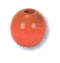(A) 79
(B) 63
(C) 69
Explanation:
and 8 green beads in a jar.
Then Sasha put 16 beads into the jar.
24 + 31 + 8 + 16 = 79
79 beads are in the jar now

TAKE HOME ACTIVITY • Have your child explain how he or she solved one of the exercises in this lesson.
We had added the two digit numbers to find the sum of numbers.

Texas Go Math Grade 2 Lesson 7.5 Homework and Practice Answer Key

Question 1.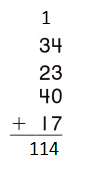Explanation:
The sum of 4 two digit numbers are added
34 + 23 + 40 + 17 = 114

Question 2.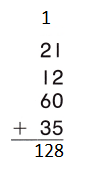Explanation:
The sum of 4 two digit numbers are added

Question 3.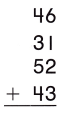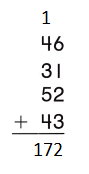Explanation:
The sum of 4 two digit numbers are added
46 + 31 + 52 + 43 = 172

Question 4.Explanation:
The sum of 4 two digit numbers are added
22 + 19 + 41 + 63 = 145

Question 5.Explanation:
The sum of 4 two digit numbers are added
34 + 10 + 37 + 55 = 136

Question 6.Explanation:
The sum of 4 two digit numbers are added
12 + 24 + 53 + 30 = 119

Problem Solving

Question 7.
Multi-Step Kate read 32 pages on Monday, 21 pages on Tuesday, and 15 pages on Wednesday. Then she read some more on Thursday. Altogether she read 88 pages. How many pages did she read on Thursday?
32 + 21 + 15 + ☐ = 88
___________ pages
20 pages
Explanation:
32 + 21 + 15 + ☐ = 88
32 + 21 + 15 + 20 = 88
20 pages that she read on Thursday

Lesson Check

Question 8.
There were 15 red marbles, 21 blue marbles, and 7 green marbles in a bag. Then Cameron put 18 black marbles into the bag. How many marbles are in the bag now?
(A) 57
(B) 61
(C) 51
Explanation:
There were 15 red marbles,
21 blue marbles,
and 7 green marbles in a bag.
Then Cameron put 18 black marbles into the bag.
15 + 21 + 7 + 18 = 61
61  marbles are in the bag now

Question 9.
There are 18 first graders, 20 second graders. and 16 third graders at the science fair. There are also 24 adults at the science fair. How many people are at the science fair?
(A) 68
(B) 54
(C) 78
Explanation:
and 16 third graders at the science fair.
There are also 24 adults at the science fair.
18 + 20 + 16 + 24 = 78
78 people are at the science fair

Question 10.
Mr. Gomez has a toy store. In one day, he sells 22 dolls and 20 cars. He also sells 18 games and 26 puzzles. How many toys does he sell?
(A) 86
(B) 76
(C) 88
Explanation:
Mr. Gomez has a toy store. In one day, he sells 22 dolls and 20 cars.
He also sells 18 games and 26 puzzles.
22 + 20 + 18 + 26 = 86
86  toys that he sell

Question 11.
Multi-Step Some friends need 100 bows for a project. Sara brings 2 bows, Angela brings 50 bows, and Nora brings 34 bows. How many more bows do they need?
(A) 6
(B) 4
(C) 5